# Fleming's Left Hand Rule

## The Motor Effect

The motor effect occurs when a conductor carrying a current is placed in a magnetic field that is caused by a secondary magnet. The magnet and the conductor exert a force on each other.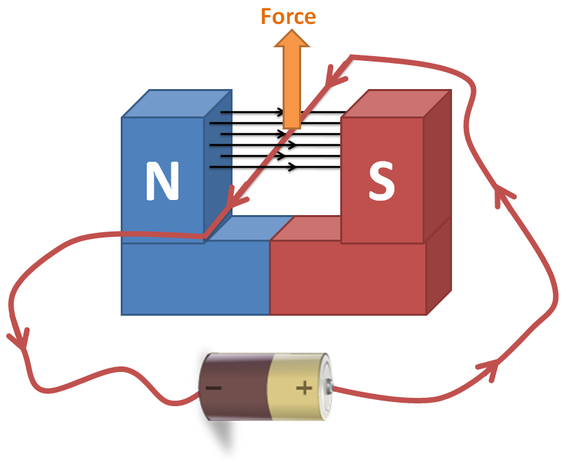Electric motors is the application of the motor effect.

A coil of wire carrying a current in a magnetic field tends to rotate.

See the following slides below: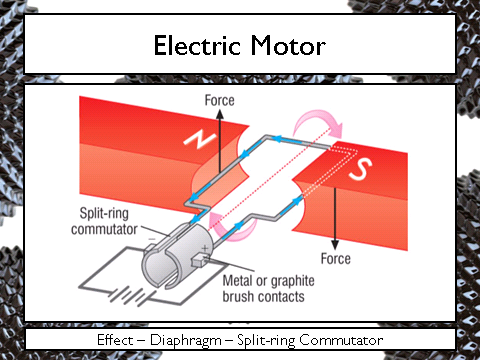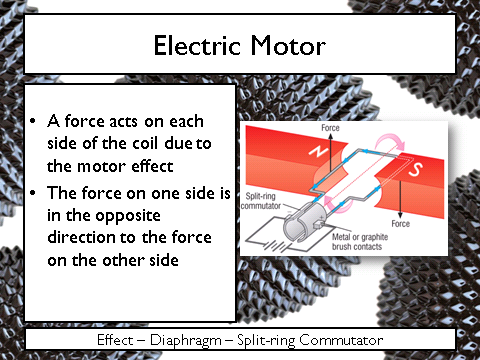﻿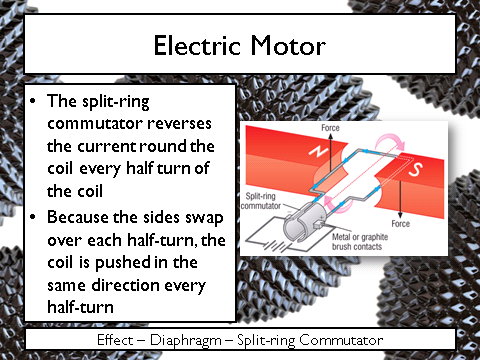If asked how an electric motor works, ensure you can write the above bullet points.

To increase the strength of an electric motor you need to:

• Increase the size of the magnets
• Increase the size of the current
• Increase the __amount of wire __inside the magnetic field

## Fleming’s Left Hand Rule

Fleming came up with a way of showing the orientation of force, current and magnetic field in relation to each other. It requires the use of your left hand.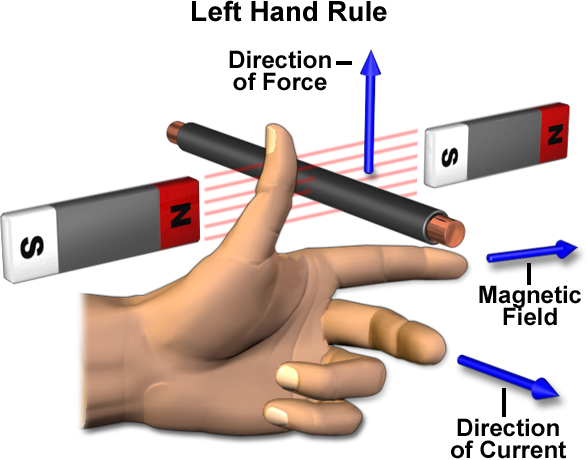Ensure that you know what the fingers and__ thumb__ represent.﻿﻿﻿

Try this: which way is the force acting?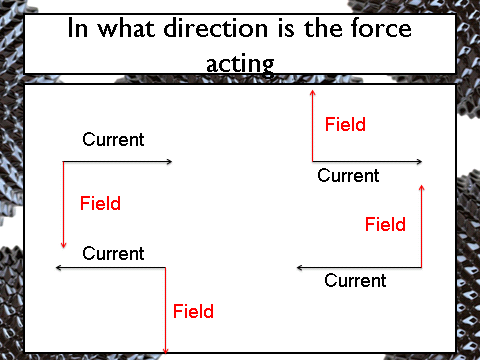Answer: into the screen

What is the effect called when a force is produced from placing a conductor inside an external magnetic field?
motor effect

## Equation

Magnetic force can be calculated via this equation which is given in your exam:

Force = Magnetic flux density x current x length of wire

F = B x I x l

• F = Force, Newtons (N)
• B = Magnetic flux density, Tesla (T)
• I = Current, Amps (A)
• l = length of wire in the magnetic field, metres (m)
In Fleming’s left hand rule, label the following in order: Thumb = ? First Finger = ? Second Finger = ?
Force Magnetic field Current